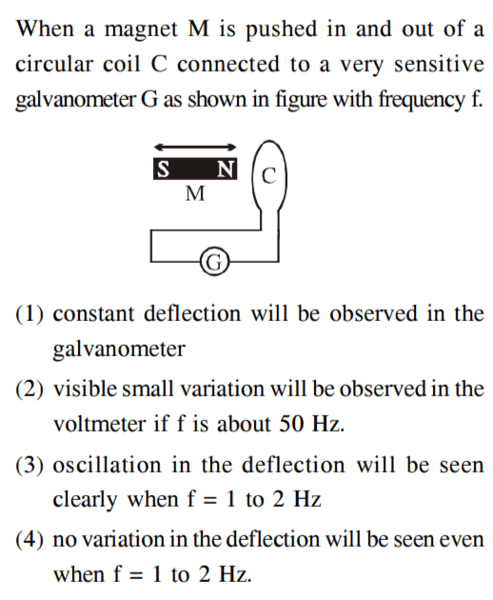# Magnet moved in a circular coil

## Homework Statement## The Attempt at a Solution

As the magnet moves in and out of the coil , flux linked with the coil changes , as a result an EMF is induced in the coil . A current flows in the coil . The current depends on how fast the magnet is moved . Current will vary in the coil . I think Options 1) and 4) can be ignored .

I don't understand how the induced current depends upon the frequency with which magnet is moved .

How do we choose between 2) and 3) ?

#### Attachments

BvU
Homework Helper
Hi,
Good work so far. Choosing between 2 and 3 means finding something that has to do with frequency. The composer (deliberately?) complicated the issue by using the term voltmeter.

What is the form of the induced EMF, as e.g. expressed in terms of a function ##V(t)## ?

Hi ,

The composer (deliberately?) complicated the issue by using the term voltmeter.

Let us assume that it is a printing mistake . Please read voltmeter as Galvanometer .

What is the form of the induced EMF

It is dΦ/dt or AdB/dt i.e area (of the coil) times rate of change of magnetic field through the coil .

BvU
Homework Helper
Yes, so quickly varying (like e.g. a sine) at 50 Hz and slowly at 1 or 2 Hz. With an amplitude proportional to the frequency. What would a galvanometer show in case 2 ? And in case 3 ?

•Jahnavi
With an amplitude proportional to the frequency.

Why ?

What would a galvanometer show in case 2

Visible large variation . Option 2) says "small" variation .

I missed out on the word "small" given in the second option .

Option 2) can also be left out .

Last edited:
BvU
Homework Helper
Why ?
The current depends on how fast the magnet is moved
So a bigger signal in 2 than in 3.

Nevertheless:
Visible large variation . Option 2) says "small" variation .
No. The amplitude of the EMF may be a lot bigger than in 3, but the point is that a) the galvanometer can't follow that so fast, and even if it could, you wouldn't be able to follow it so fast.

•Jahnavi
The amplitude of the EMF may be a lot bigger than in 3, but the point is that a) the galvanometer can't follow that so fast, and even if it could, you wouldn't be able to follow it so fast.

Sorry .

Do you mean there will be a large variation in case of option 2) but it might not be visible . Is "visible" the issue in option 2) ?

The needle of Galvanometer will move to and fro from one side to other very quickly but it will be visible . I might be wrong .

BvU
Homework Helper
50 Hz can't be followed by a mechanical galvanometer. So there won't be a large oscillation.

A modern intelligent multimeter would probably detect an AC signal and use a rectifier -- and show a voltage.

My money is on 3) for this exercise ('very sensitive galvanometer')

•Jahnavi
50 Hz can't be followed by a mechanical galvanometer. So there won't be a large oscillation.

So , the needle of the Galvanometer more or less stays in its place ?

BvU
•